## General Chemistry

We have seen earlier that galvanic cells have a positive cell potential (negative Gibbs free energy) which makes them spontaneous. This means that, just like for any other process, the reverse reaction of any spontaneous redox reaction will be nonspontaneous as it will have a negative cell potential and a positive Gibbs free energy.

For example, the reaction between sodium and chlorine is violent as it is very exothermic and therefore, we can also predict that the overall cell potential will be a positive value.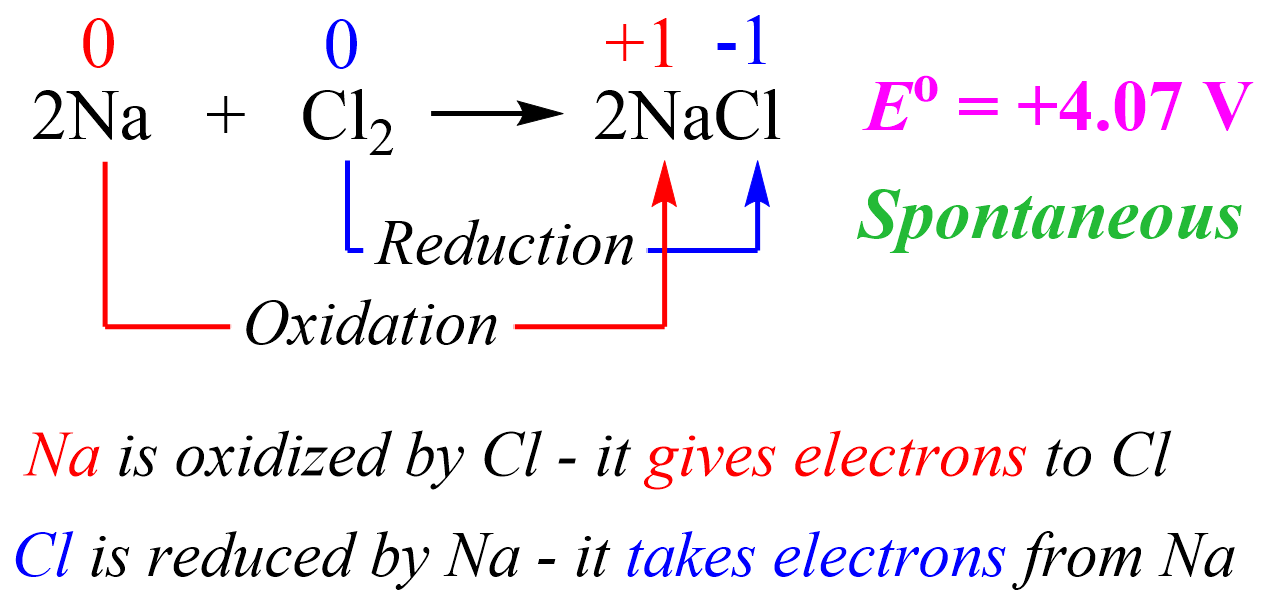The cell potential is estimated based on the half-reactions under standard conditions.

We can explain this reaction simply based on the fact that Na is a metal and likes to lose an electron to become a cation which is easily achieved when there is a nonmetal with great electronegativity such as Cl. So, in a simple scheme, we can visualize this as Cl pulling the electron from Na because it likes electrons a lot more than Na does.

Now, here is the question: if the reverse reaction is nonspontaneous, (how) can we force it to occur? In the case of Na and Cl2, the target reverse reaction would be: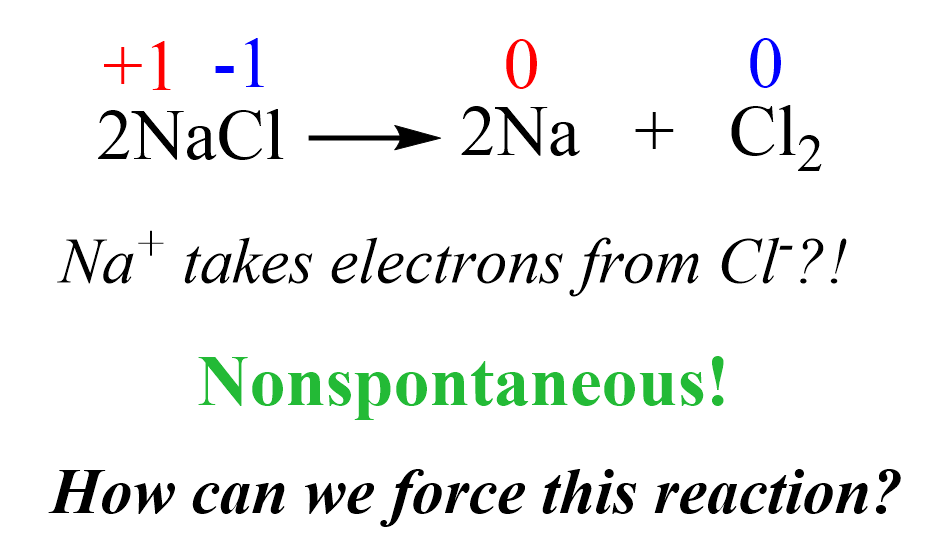Can we make Cl ions give the electrons back to Na+ and prepare Na metal and Cl2 gas?

Remember, from thermodynamics that if a process is endothermic (more accurately endergonic if we are talking of the Gibbs free energy), then we need to supply the necessary amount of energy to push it forward: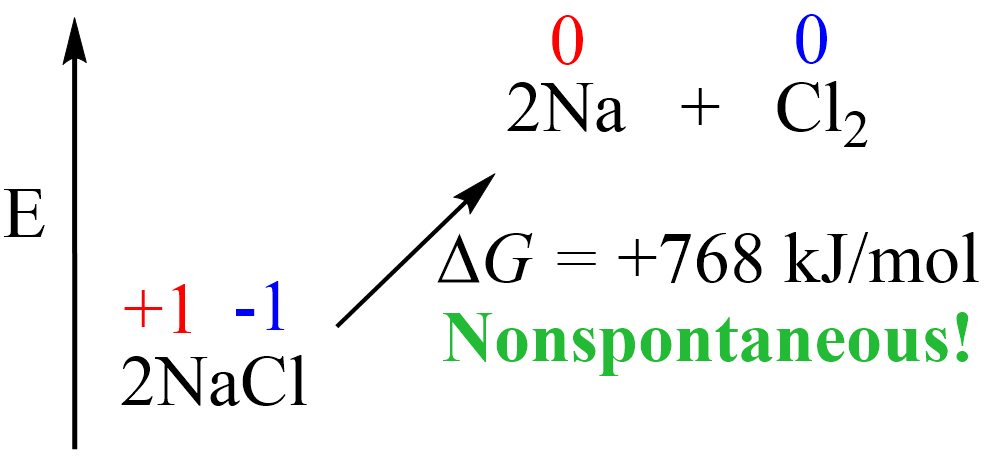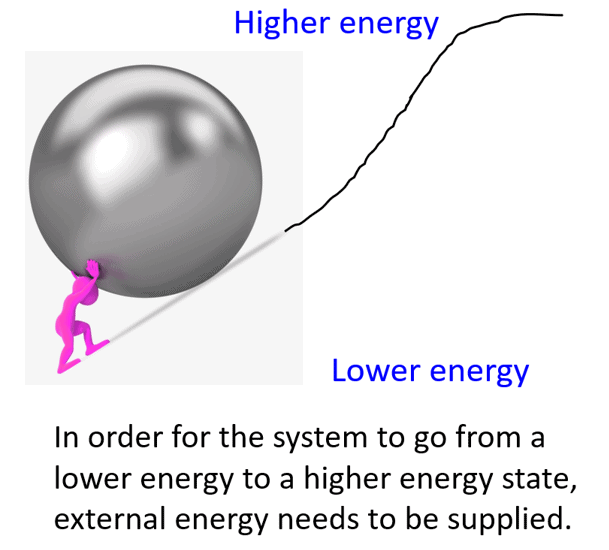The same principle applies here: if we want NaCl to convert into Na and Cl2, we must provide the necessary energy, which in other words, would mean pushing the electrons in the opposite direction by taking them from Cl ions and giving them to Na+ ions.

It turns out that reversing the direction of a redox reaction is possible if external electrical power is used. In this case, we have an electrolytic cell where electrical current drives an otherwise nonspontaneous redox reaction through a process called electrolysis. So, let’s break down this process to gain a better understating using the sample of NaCl.

# Electrolysis of Molten NaCl

The first thing we will need to do electrolysis of NaCl is a battery with an estimated potential of greater than 4.07 V as this was the estimated cell potential of the reaction between Na and Cl2. This cell potential is simply a qualitative description to emphasize that the reverse reaction will be nonspontaneous. Why qualitative? One reason would be the fact that we are going to need molten NaCl which is possible only at 800 oC and that is far from standard conditions.

Molten NaCl is needed because if we use a solution of NaCl, there is also the possibility of the water undergoing electrolysis.

We will discuss the electrolysis of aqueous NaCl as well, but for now, let’s draw what we have so for the electrolysis of molten NaCl:The electrode on the left side is connected to the positive end of the battery and therefore, it is positively charged, and the one on the right side is negatively charged.

Notice that the electrodes themselves are not part of the reactions and therefore, unlike galvanic cells, the electrodes of an electrolytic cell are typically made of the same metal.

So, what is going to happen with the Na+ and Cl ions in the presence of these electrodes?

Because opposite charges attract, Na+ ions are going to accumulate at the negatively charged electrode, and the Cl ions will surround the positively charged electrode: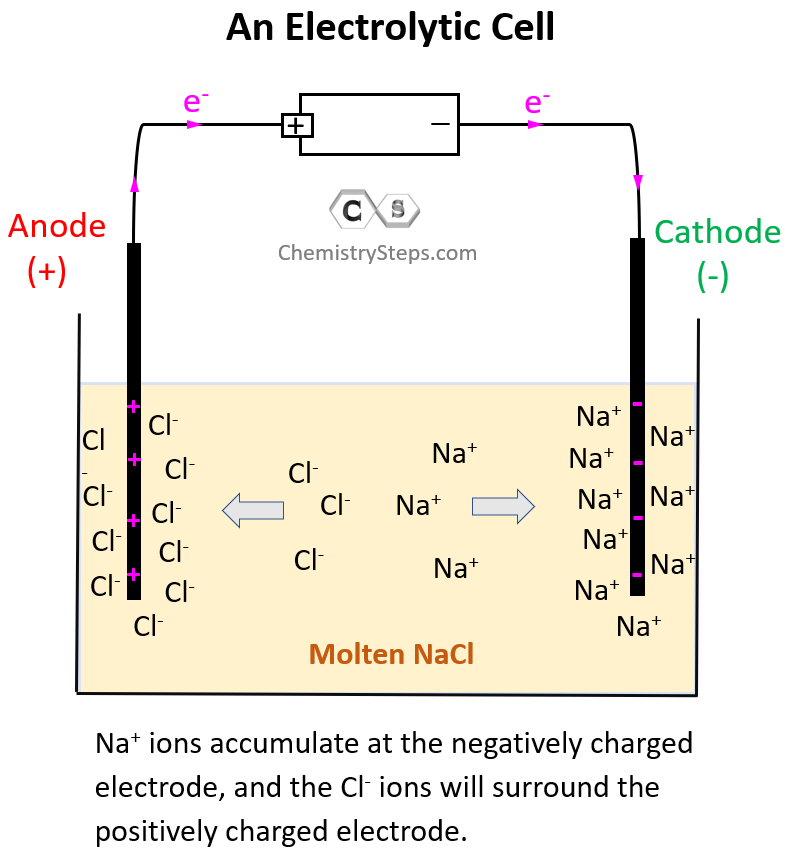Now, the negatively charged electrode is going to push electrons to the Na+ ions thus reducing them (cathode) to Na metal. On the other hand, the positively charged electrode needs electrons it is going to pull them from the Cl ions despite the fact that they do not want to give them. This is the oxidation half-reaction and therefore, the electrode is the anode. The key here is the difference in the power of pulling the electrons and since the battery has a greater potential than the reaction between Na and Cl2, it does take the electrons from Cl ions:So, on the left electrode, Cl ions are being oxidized to Cl2, and therefore, this is the anode, while the Na+ ions are being reduced on the right side electrode making it the cathode.

Remember, regardless of the type of electrochemical cell, oxidation always occurs on the anode, and the reduction occurs on the cathode.

Notice, however, that the anode is positively charged here unlike what we saw in galvanic cells, where it was considered to be negatively charged as it gave the electrons to the cathode thus undergoing oxidation. In electrolytic cells, the external power source draws the electrons from the anode thus making it positive. So, keep in mind:

• In a voltaic cell, the anode is negative as it takes the electrons from the cathode and thus making positive.
• In an electrolytic cell, the anode is positive as the external battery takes the electrons from it, and provides them to the cathode making it negative.

Once again, in both cases, oxidation always takes place on the anode, and the reduction takes place on the cathode.

So, let’s write down each half-reaction. On the anode, we have the oxidation of Cl to Cl2, and on the cathode, we have the reduction of Na+ to Na:

Anode (oxidation): 2 Cl(l) → Cl2(g)  + 2 e–

Cathode (reduction): 2Na+(l) + 2e- → 2Na(s)

The overall reaction then will be written as:

2Na+(l) + 2 Cl(l) → 2Na(s) + Cl2(g

# Other Electrolysis Reactions

The electrolysis of NaCl is by far the most common example you will see in textbooks and during lectures, however, the principle of electrolysis can be applied to any nonspontaneous redox reaction. For example, Cu2+ ions can oxidize Sn to Sn2+ because of their higher reduction potential (greater tendency to be reduced) than Sn2+:

Sn(s) + Cu2+(aq) → Sn2+(aq) + Cu(s)

The values of half-reactions can be found in a standard textbook, and to find the cell potential, we simply add them after switching the sign for the reverse reaction.

Sn(s) → Sn2+(aq) + 2e–  E= +0.14 V

Cu2+(aq) + 2e–  → Cu(sE= +0.34 V
______________________________________

Sn(s) + Cu2+(aq) → Sn2+(aq) + Cu(sE= +0.48 V

Feel free to check this article for more details on calculating the standard cell potential.

Now, if we set up an electrolytic cell, we can force the reverse reaction where Sn2+ ions oxidize Cu to Cu2+ ions granted the external voltage is higher than 0.48 V. With that said, it is not really Sn2+ that oxidizes Cu, but rather the external voltage. The metals and their electrolyte simply support the current flow by providing and accepting the electrons.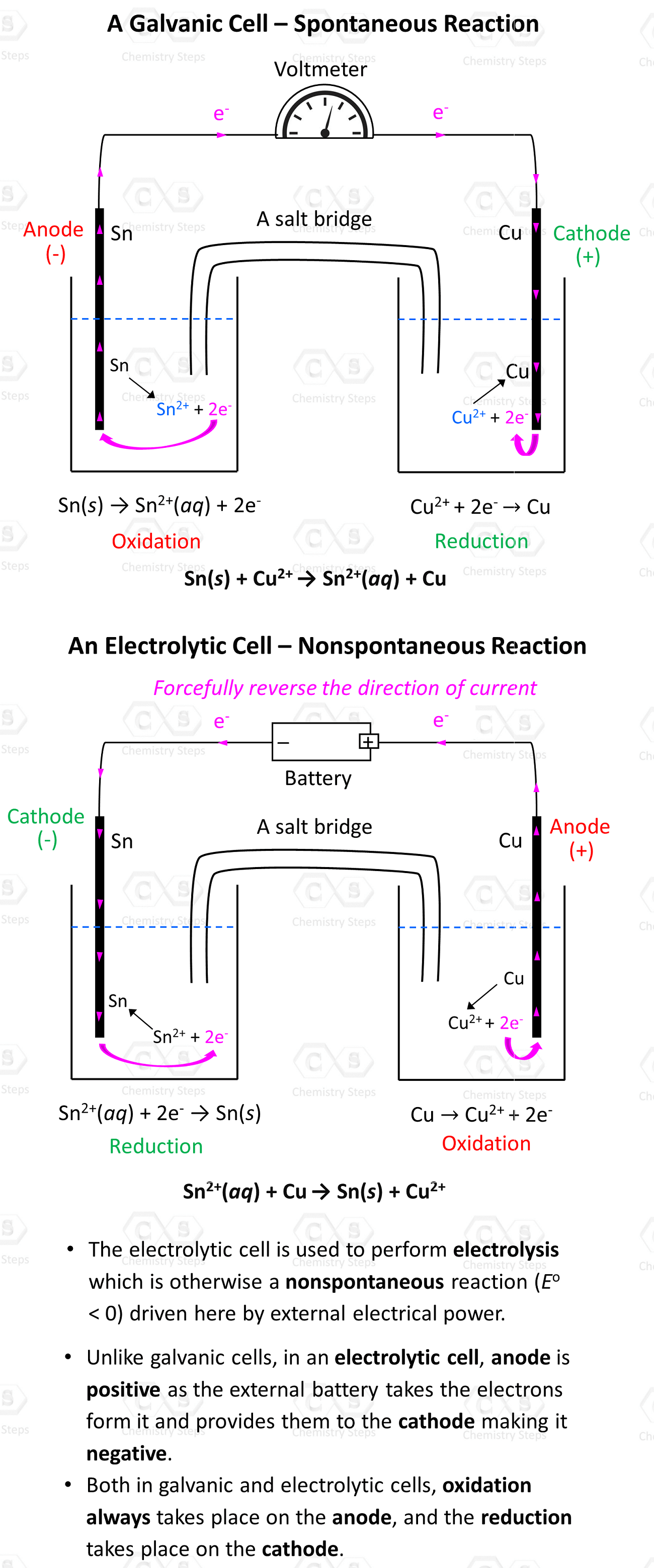# Electrolysis of Aqueous NaCl

Now that we have gone over the principle of electrolysis, let’s discuss perhaps a more confusing example which is the electrolysis of NaCl in an aqueous solution. The difference here compared to the electrolysis of molten sodium chloride is that the water can also be oxidized and/or reduced when external power is provided according to the following half-reactions:

Oxidation (anode): 2 H2O(l) → O2(g) + 4 H+(aq) + 4 e   E° = -1.23 V

Reduction (cathode): 2 H2O(l) + 2 e → H2(g)  + 2 OH(aq)    E° = -0.83 V

Note: The cell potentials given above are for standard conditions which imply 1 M H+. However, at pH 7, [H+] = [OH] = 10-7 M, so we do not have standard conditions, and the cell potentials are 0.82 V and -0.41 V respectively.

Now, each of these reactions is going to compete with the oxidation and reduction reactions that we saw in the electrolysis of molted NaCl. Let’s recall: Cl ions were oxidized to Cl2 according to the following equation:

2 Cl(aq) → Cl2(g)  + 2 e

And Na+ ions were reduced as they accept the electrons from the Cl ions:

Na+(aq) + e- → Na(s)

So, the two possible oxidation reactions in the NaCl solution would be:

2 H2O(l) → O2(g) + 4 H+(aq) + 4 e   E° = -1.23 V

2 Cl(aq) → Cl2(g)  + 2 e–    E° = -1.36 V

Based on the higher cell potential, we’d expect water to be oxidized easier than Cl. Interestingly though, it was found that Oxygen gas is not formed at the anode, and instead, chlorine gas is produced. How can we explain this? The answer is what’s called overvoltage or overpotential which is the difference between the electrode potential and the actual voltage required to cause electrolysis. The need for overpotential is caused by the slow electron transfer rate at the electrode–solution interface thus limiting the amount of current passing through an electrolytic cell. It turns out that the overpotential is higher for the formation of oxygen compared to that of chlorine and therefore, the oxidation of Cl ions producing Cl2 is what occurs at the anode.

Overpotential often makes it difficult to predict with certainty which of the possible half-reactions with close E° values will occur, and only experiments can show what actually happens.

So, this was about the oxidation at the anode. The two possible reduction reactions at the cathode would be:

Na+(aq) + e- → Na(sE° = -2.71 V

2 H2O(l)  + 2 e → H2(g)  + 2 OH(aqE° = -0.83 V

The Eo values are quite different, and as expected, because of a higher (less largely negative) standard reduction potential, water is reduced preferentially producing bubbles of hydrogen gas at the cathode.

Therefore, by adding the two half-reactions, we can obtain the overall electrolysis reaction:

Anode (oxidation): 2 Cl(aq) → Cl2(g)  + 2 e–    E° = -1.36 V

Cathode (reduction): 2 H2O(l) + 2 e → H2(g)  + 2 OH(aqE° = -0.83 V

______________________________________________________________

2 Cl(aq) + 2 H2O(l)  + 2 e → Cl2(g)  + 2 e  + H2(g)  + 2 OH(aq)

Overall cell reaction: 2 Cl(aq) + 2 H2O(l) → Cl2(g) + H2(g)  + 2 OH(aq) E° = -2.19 V

This shows that, unlike in the electrolysis of molten NaCl, the Na+ ions are simply spectator ions and do not participate in the electrolysis of aqueous NaCl.

To summarize, remember that electrolysis is performed in an electrolytic cell, and it is the process of driving a nonspontaneous redox reaction by using external electrical power.

• Both in galvanic and electrolytic cells, oxidation always takes place on the anode, and the reduction takes place on the cathode.
• In a voltaic cell, the anode is negative as it takes the electrons from the cathode and thus making positive.
• In an electrolytic cell, the anode is positive as the external battery takes the electrons from it and provides them to the cathode making it negative.

Electrolysis also has numerous other applications. For example, fluorine cannot be prepared by any spontaneous chemical reaction. It was found in 1886 by the French chemist Henri Moissan that passing electrical current through a molten mixture of potassium fluoride and hydrogen fluoride produces Fluorine, and it is still prepared commercially by the same method. Another application of electrolysis is the preparation of metals from their oxides which is how most metals are found in Earth’s crust.

In the next article, we will discuss the electrolysis of water.